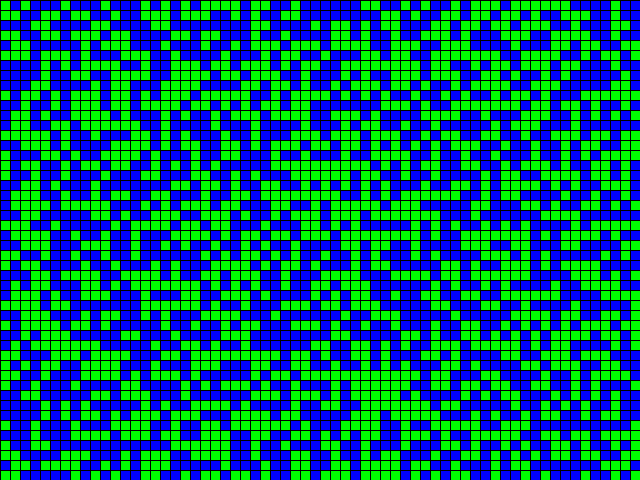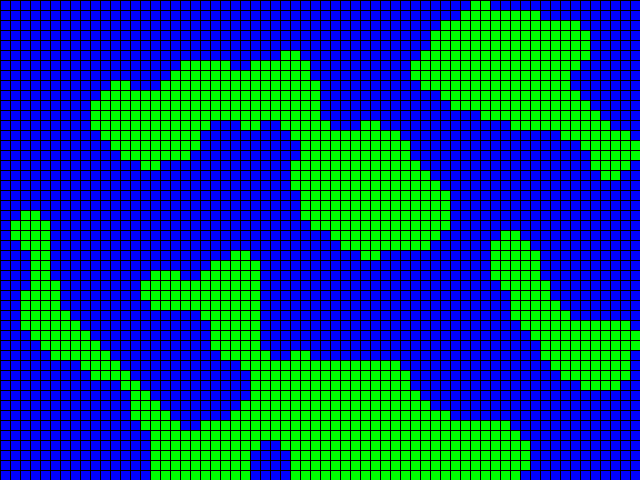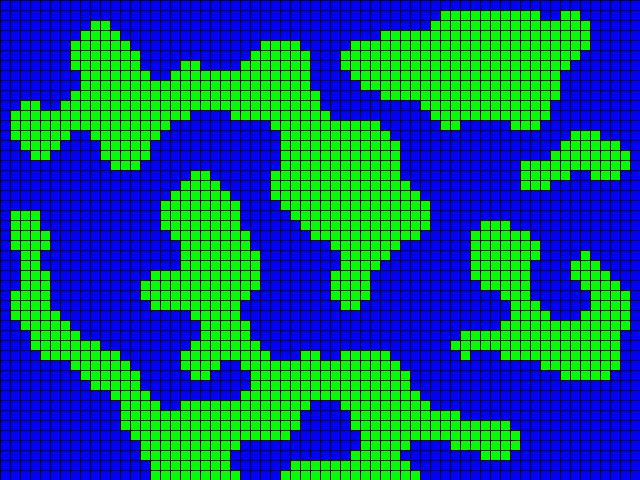# Using cellular automata to generate random land and water maps with Flash

After the first attempt to generate land and water maps using random numbers, it’s time to try to do the same thing using cellular automata.

A cellular automaton (plural: cellular automata) is a discrete model studied in computability theory, mathematics, physics, complexity science, theoretical biology and microstructure modeling.

It consists of a regular grid of cells, each in one of a finite number of states, such as “On” and “Off” (or “Land” and “Water”).

The grid can be in any finite number of dimensions. For each cell, a set of cells called its neighborhood (usually including the cell itself) is defined relative to the specified cell.

For example, the neighborhood of a cell might be defined as the set of cells a distance of 2 or less from the cell.

An initial state (time t=0) is selected by assigning a state for each cell. A new generation is created (advancing t by 1), according to some fixed rule (generally, a mathematical function) that determines the new state of each cell in terms of the current state of the cell and the states of the cells in its neighborhood.

For example, the rule might be that the cell is “On” in the next generation if exactly two of the cells in the neighborhood are “On” in the current generation, otherwise the cell is “Off” in the next generation.

Typically, the rule for updating the state of cells is the same for each cell and does not change over time, and is applied to the whole grid simultaneously, though exceptions are known (source).

What does this mean? In simple words, we are placing random land and water tiles in a map, and let the cellular automaton (read carefully: “automaton” not “automation”) draw the map according to a set of predefined rules.

So this is an example of a starting map, generated with random tiles of water and land, with each tile having the same probability of being land or water:Once we have this map, we apply the following rule for a given number of iterations, set to 8 in the example:

If a land tile is surrounded by at least four land tiles (diagonals included), it remains a land tile, otherwise it’s converted into a water tile. If a water tile is surrounded by at least five land tiles (diagonals included), it’s converted into a land tile, otherwise it remains a water tile.

Now the only last thing to define is whether to apply the rule tile by tile or simultaneously. It’s up to you, anyway, this is what we get starting from the initial map applying eight times the rule tile by tile:And this is what happens when applying the rule simultaneously.Comparing with the maps generated in the previous example, these maps are much more realistic and smooth.

Finally, here it is the source code:

```package {
import flash.display.Sprite;
import flash.events.MouseEvent;
public class terrain extends Sprite {
private const WATER:int=0;
private const LAND:int=1;
private var iterations:int=8;
private var simultaneously:Boolean=false;
private var landRatio:Number=0.50;
private var mapWidth:int=64;
private var mapHeight:int=48;
private var tileSize:int=10;
private var canvas:Sprite;
private var landArray:Array;
private var newLandArray:Array;
public function terrain() {
canvas = new Sprite();
landArray=new Array();
newLandArray=new Array();
generateMap();
}
private function generateMap():void {
landOrWater();
drawMap();
}
private function drawMap():void {
for (var i:int=0; i=5) {
if (! simultaneously) {
landArray[i][j]=land;
} else {
newLandArray[i][j]=land;
}
} else {
if (! simultaneously) {
landArray[i][j]=defaultLand;
} else {
newLandArray[i][j]=defaultLand;
}
}
}
}
}
if (simultaneously) {
for (i=0; i
And this is the result:

Click on the map to generate a new one. No need to download anything since you just have to copy/paste the code into the one you find in this post.
Next time, I'll explain the source code and we'll see how to generate these maps in a given sequence.
```
214 GAME PROTOTYPES EXPLAINED WITH SOURCE CODE
// 1+2=3
// 10000000
// 2 Cars
// 2048
// Avoider
// Ballz
// Block it
// Blockage
// Bloons
// Boids
// Bombuzal
// Breakout
// Bricks
// Columns
// CubesOut
// Dots
// DROP'd
// Dudeski
// Eskiv
// Filler
// Fling
// Globe
// HookPod
// Hundreds
// InkTd
// Iromeku
// Lumines
// Magick
// MagOrMin
// Maze
// Memdot
// Nano War
// Nodes
// o:anquan
// Ononmin
// Pacco
// Phyballs
// Platform
// Poker
// Pool
// Poux
// Pudi
// qomp
// Racing
// Renju
// SameGame
// Security
// Sling
// Slingy
// Sokoban
// Splitter
// Sproing
// Stack
// Stringy
// Sudoku
// Tetris
// Threes
// Toony
// Turn
// TwinSpin
// vvvvvv
// Wordle
// Worms
// Yanga
// Zhed
// zNumbers